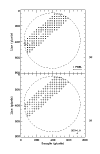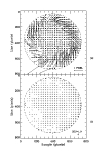Next: 5.4 Evaluation of the Up: 5.3 Pattern Matching Algorithm Previous: 5.3 Pattern Matching Algorithm

5.3.1 Intermediate CROSS-CORR Output

The intermediate results from CROSS-CORR can be used to monitor the geometric/photometric state of the raw science image. In the vector displacements (VD) file, these intermediate results are stored in a binary table extension containing the following output for each sampling position:

1.
science image x-position (sample),
2.
science image y-position (line),
3.
ITF x-position of best match (sub-pixel accuracy),
4.
ITF y-position of best match (sub-pixel accuracy),
5.
maximum cross-correlation coefficient (computed for pixel location accuracy),
6.
number of pixels used to calculate the correlation, and
7.
the ITF level used for CROSS-CORR
Items 1 and 2 are the pre-defined fiducial locations about which CROSS-CORR performs its search. Items 3 and 4 are the best match positions between the raw science image and the ITF image. Note that these final positions are in quantized units of 1/8 pixel, which is a consequence of the design of CROSS-CORR. Item 5 is the maximum correlation coefficient which can range between 0.0 and 1.0. In practice the correlations range between -1.0 and +1.0, but negative correlations have been deemed invalid. Item 6 is the number of points used to compute the correlation; this will range from 139-529 pixels for a 23 × 23 pixel template. Item 7 is the ITF level used for the correction and will range in value from 1 to 12.

The total number of entries in the binary table extension is both camera and dispersion dependent. Table 5.1 contains the number of fiducial locations used in CROSS-CORR.

 Number of Locations Camera Low Dispersion High Dispersion LWP 150 490 LWR 151 511 SWP 139 510

The data contained in the binary table extension can be used to produce diagnostic plots for CROSS-CORR. Panel a of Figure 5.1 shows the displacements computed between an SWP low-dispersion image and the SWP ITF. The small boxes denote the fiducial locations where a successful correlation was determined. The associated vector represents the computed displacement between the raw science image and the ITF; the vector displacements have been magnified for visual clarity. Single points mark the locations of fiducials with unsuccessful correlations. Panel b of Figure 5.1 shows the magnitude of the correlation coefficients corresponding to the results in Panel a. Larger plus signs denote stronger correlations. The dashed lines represent the region of the image that is photometrically corrected. Recall that all of these correlations have passed the 1% probability distribution test which means that there is less than a 1% probability any of these correlations could have arisen by random chance. The corresponding example for high-dispersion data is illustrated in FigureFigure 5.1:  (a) Displacements computed between an SWP low-dispersion image and the SWP ITF. (b) Magnitude of the correlation coefficients corresponding to the results shown in Panel a.Figure 5.2: (a) Displacements computed between an SWP high-dispersion image and the SWP ITF. (b) Magnitude of the correlation coefficients corresponding to the results shown in Panel a.Next: 5.4 Evaluation of the Up: 5.3 Pattern Matching Algorithm Previous: 5.3 Pattern Matching Algorithm
Karen Levay
12/4/1997